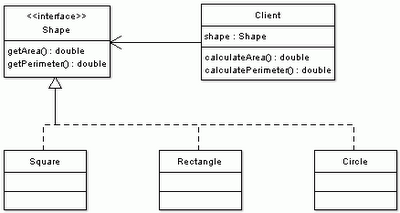## Saturday, August 19, 2006

### Swich Statement code smell and Polymorphism

One of the symptoms of object-oriented programming is the lack of `switch` or `case` statements. Imagine that we have some client class that calculates the area and perimeter of particular geometrical shapes.

``public class Client { private double a; private double b; private double r; ... public double calculateArea(int shape) {     double area = 0;     switch(shape) {         case SQUARE:             area = a * a;             break;         case RECTANGLE:             area = a * b;             break;         case CIRCLE:             area = Math.PI * r * r;             break;     }     return area; } public double calculatePerimeter(int shape) {     double perimeter = 0;     switch(shape) {         case SQUARE:             perimeter = 4 * a;             break;         case RECTANGLE:             perimeter = 2 * (a + b);             break;         case CIRCLE:             perimeter = 2 * Math.PI * r;             break;     }     return perimeter; } ...}``

The previous code is clearly a poor design that limits the current client to only work with three types of shapes. The problem with `switch` statements is the duplication and that is a code smell. There are usually several places in the code where the behaviour slightly deviates and these `switch` statements are present (e.g. in `calculateArea` and `calculatePerimeter` methods). Even worse case is if we work with objects where the `switch` is replaced by multiple `instanceof` conditions.

``public class Client { ... public double calculateArea(Object shape) {     double area = 0;     if (shape instanceof Square) {         Square square = (Square) shape;         area = square.getA() * square.getA();     }     else if (shape instanceof Rectangle) {         Rectangle rectangle = (Rectangle) shape;         area = rectangle.getA() * rectangle.getB();     }     else if (shape instanceof Circle) {         Circle circle = (Circle) shape;         area = Math.PI * cirle.getR() * cirle.getR();     }     return area; } public double calculatePerimeter(Object shape) {     double perimeter = 0;     if (shape instanceof Square) {         Square square = (Square) shape;         perimeter = 4 * square.getA();     }     else if (shape instanceof Rectangle) {         Rectangle rectangle = (Rectangle) shape;         perimeter = 2 * (rectangle.getA() + rectangle.getB());     }     else if (shape instanceof Circle) {         Circle circle = (Circle) shape;         perimeter = 2 * Math.PI * cirle.getR();     }     return perimeter; }}``

To improve the desing we make `Square`, `Rectangle` and `Circle` have a commont root. By that I mean that they either extend the same class, such as `AbstractShape` or that they implement a common interface, such as `Shape` or ideally both.Then we can eliminate the switch statement code smell very easily. We replace it with polymorphism.

``Shape.java filepublic interface Shape { public double getArea(); public double getPerimeter();}Square.java filepublic class Square implements Shape { private double a; ... public double getArea() {     return a * a; } public double getPerimeter() {     return 4 * a; }}Rectangle.java filepublic class Rectangle implements Shape { private double a; private double b; ... public double getArea() {     return a * b; } public double getPerimeter() {     return 2 * (a + b); }}Circle.java filepublic class Circle implements Shape { private double r; ... public double getArea() {     return Math.PI * r * r; } public double getPerimeter() {     return 2 * Math.PI * r; }}``

And such refactoring simplifies the `Client` code. It also allows for easy extensibility by implementations of other shapes without changing a single line of `Client` code.

``public class Client { private Shape shape; ... public double calculateArea() {     return shape.getArea(); } public double calculatePerimeter() {     return shape.getPerimeter(); }}``

Another way of looking at it is from the responsibility point of view. Why should the client be responsible for calculating the area or the perimeter; or have a knowledge about shape's internals (e.g. number of sides, radius, etc.) It is the responsibility of each shape and all that the client needs to know is that a shape has a perimeter and area.

To sum this all up:Anonymous said...

Obviously a programming genius.
if you implement an interface , each shape has to provide functions of the interface.

so how many sides does a circle have?
and whats the radius of a square?

Unknown said...

Dude, inheritance is good if your objects have something in common (e.g. all shapes have an area).

Obviously, inheritance is not suitable for everything, it isn't a silver bullet to code re-use.

If you are interested in specifics of each implementation or your objects do not have much in common you'll need to use something else.

Rob Meyer said...

I think the point here is that a switch statement (pretty much) always represents some conceptual commonality. For anonymous, that means that you don't put GetNumberOfSides() or GetRadius() into the common interface. You also wouldn't write a method like this:

switch(shape.Type)
Type.Circle:
Type.Square:
// What happens here?

You can implement as many interfaces as you want, so if something represents a first class concept in your conceptual model, no reason not to elevate it to an interface. Dushan is right of course that it's likely unmanageable to create a single interface for every switch statement, but finding the ones that make sense for your application to avoid switch or big if/then blocks is why design isn't easy.Anonymous said...

Hey,

Thanks for this - Someone told me they were asked this at interview so it's a good one to know!

Unknown said...

So how do you fix this?

if (obj instanceof String) {
// it's a String
} else if (obj instanceof Integer) {
// it's an Integer
}